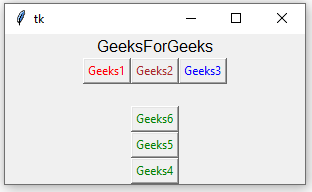GFG App
Open AppBrowser
Continue

# Python Tkinter – Frame Widget

Python offers multiple options for developing GUI (Graphical User Interface). Out of all the GUI methods, tkinter is the most commonly used method. It is a standard Python interface to the Tk GUI toolkit shipped with Python. Python with tkinter is the fastest and easiest way to create the GUI applications. Creating a GUI using tkinter is an easy task.

## Frame

A frame is a rectangular region on the screen. A frame can also be used as a foundation class to implement complex widgets. It is used to organize a group of widgets.

Syntax:
The syntax to use the frame widget is given below.

`w = frame( master, options)`

Parameters:

• master: This parameter is used to represents the parent window.
• options:There are many options which are available and they can be used as key-value pairs separated by commas.

Options:
Following are commonly used Option can be used with this widget :-

• bg: This option used to represent the normal background color displayed behind the label and indicator.
• bd: This option used to represent the size of the border around the indicator and the default value is 2 pixels.
• cursor: By using this option, the mouse cursor will change to that pattern when it is over the frame.
• height: The vertical dimension of the new frame.
• highlightcolor: This option used to represent the color of the focus highlight when the frame has the focus.
• highlightthickness: This option used to represent the color of the focus highlight when the frame does not have focus.
• highlightbackground: This option used to represent the thickness of the focus highlight..
• relief: The type of the border of the frame. It’s default value is set to FLAT.
• width: This option used to represents the width of the frame.

Example:

 `from` `tkinter ``import` `*` `root ``=` `Tk() ` `root.geometry(``"300x150"``) ` ` `  `w ``=` `Label(root, text ``=``'GeeksForGeeks'``, font ``=` `"50"``)  ` `w.pack() ` ` `  `frame ``=` `Frame(root) ` `frame.pack() ` ` `  `bottomframe ``=` `Frame(root) ` `bottomframe.pack( side ``=` `BOTTOM ) ` ` `  `b1_button ``=` `Button(frame, text ``=``"Geeks1"``, fg ``=``"red"``) ` `b1_button.pack( side ``=` `LEFT) ` ` `  `b2_button ``=` `Button(frame, text ``=``"Geeks2"``, fg ``=``"brown"``) ` `b2_button.pack( side ``=` `LEFT ) ` ` `  `b3_button ``=` `Button(frame, text ``=``"Geeks3"``, fg ``=``"blue"``) ` `b3_button.pack( side ``=` `LEFT ) ` ` `  `b4_button ``=` `Button(bottomframe, text ``=``"Geeks4"``, fg ``=``"green"``) ` `b4_button.pack( side ``=` `BOTTOM) ` ` `  `b5_button ``=` `Button(bottomframe, text ``=``"Geeks5"``, fg ``=``"green"``) ` `b5_button.pack( side ``=` `BOTTOM) ` ` `  `b6_button ``=` `Button(bottomframe, text ``=``"Geeks6"``, fg ``=``"green"``) ` `b6_button.pack( side ``=` `BOTTOM) ` ` `  `root.mainloop() `

Output:My Personal Notes arrow_drop_up